# 12.7 Magnetism in matter  (Page 6/13)

 Page 6 / 13

A solenoid with an iron core is 25 cm long and is wrapped with 100 turns of wire. When the current through the solenoid is 10 A, the magnetic field inside it is 2.0 T. For this current, what is the permeability of the iron? If the current is turned off and then restored to 10 A, will the magnetic field necessarily return to 2.0 T?

Three long, straight, parallel wires, all carrying 20 A, are positioned as shown in the accompanying figure. What is the magnitude of the magnetic field at the point P ?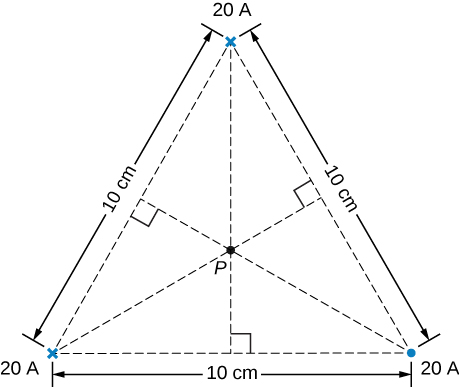$B=6.93\phantom{\rule{0.2em}{0ex}}×\phantom{\rule{0.2em}{0ex}}{10}^{-5}\text{T}$

A current I flows around a wire bent into the shape of a square of side a . What is the magnetic field at the point P that is a distance z above the center of the square (see the accompanying figure)?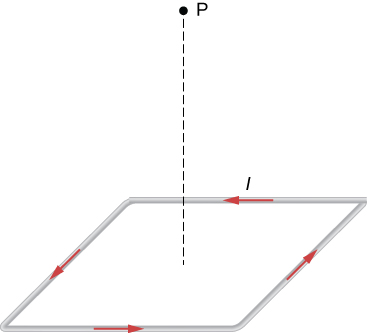The accompanying figure shows a long, straight wire carrying a current of 10 A. What is the magnetic force on an electron at the instant it is 20 cm from the wire, traveling parallel to the wire with a speed of $2.0\phantom{\rule{0.2em}{0ex}}×\phantom{\rule{0.2em}{0ex}}{10}^{5}\text{m/s?}$ Describe qualitatively the subsequent motion of the electron.$3.2\phantom{\rule{0.2em}{0ex}}×\phantom{\rule{0.2em}{0ex}}{10}^{\text{−19}}N$ in an arc away from the wire

Current flows along a thin, infinite sheet as shown in the accompanying figure. The current per unit length along the sheet is J in amperes per meter. (a) Use the Biot-Savart law to show that $B={\mu }_{0}J/2$ on either side of the sheet. What is the direction of $\stackrel{\to }{B}$ on each side? (b) Now use Ampère’s law to calculate the field.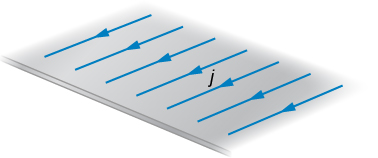(a) Use the result of the previous problem to calculate the magnetic field between, above, and below the pair of infinite sheets shown in the accompanying figure. (b) Repeat your calculations if the direction of the current in the lower sheet is reversed.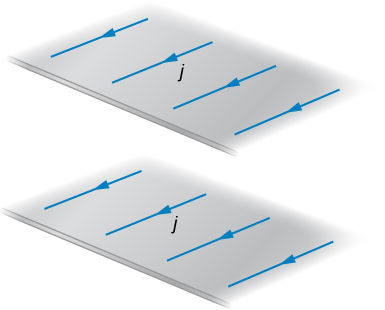a. above and below $B={\mu }_{0}j,$ in the middle $B=0;$ b. above and below $B=0,$ in the middle $B={\mu }_{0}j$

We often assume that the magnetic field is uniform in a region and zero everywhere else. Show that in reality it is impossible for a magnetic field to drop abruptly to zero, as illustrated in the accompanying figure. ( Hint : Apply Ampère’s law over the path shown.)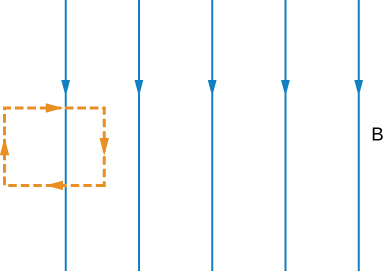How is the percentage change in the strength of the magnetic field across the face of the toroid related to the percentage change in the radial distance from the axis of the toroid?

$\frac{dB}{B}=-\phantom{\rule{0.05em}{0ex}}\frac{dr}{r}$

Show that the expression for the magnetic field of a toroid reduces to that for the field of an infinite solenoid in the limit that the central radius goes to infinity.

A toroid with an inner radius of 20 cm and an outer radius of 22 cm is tightly wound with one layer of wire that has a diameter of 0.25 mm. (a) How many turns are there on the toroid? (b) If the current through the toroid windings is 2.0 A, what is the strength of the magnetic field at the center of the toroid?

a. 52778 turns; b. 0.10 T

A wire element has $d\stackrel{\to }{l},Id\stackrel{\to }{l}=JAdl=Jdv,$ where A and dv are the cross-sectional area and volume of the element, respectively. Use this, the Biot-Savart law, and $J=nev$ to show that the magnetic field of a moving point charge q is given by:
$\stackrel{\to }{B}=\frac{{\mu }_{0}}{4\pi }\phantom{\rule{0.2em}{0ex}}\frac{qv\phantom{\rule{0.2em}{0ex}}×\phantom{\rule{0.2em}{0ex}}\stackrel{^}{r}}{{r}^{2}}$

A reasonably uniform magnetic field over a limited region of space can be produced with the Helmholtz coil, which consists of two parallel coils centered on the same axis. The coils are connected so that they carry the same current I . Each coil has N turns and radius R , which is also the distance between the coils. (a) Find the magnetic field at any point on the z -axis shown in the accompanying figure. (b) Show that dB / dz and ${d}^{2}B}{d{z}^{2}}$ are both zero at z = 0. (These vanishing derivatives demonstrate that the magnetic field varies only slightly near z = 0.)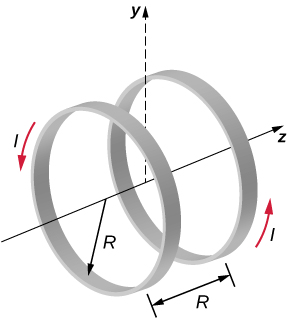${B}_{1}\left(x\right)=\frac{{\mu }_{0}I{R}^{2}}{2{\left({R}^{2}+{z}^{2}\right)}^{3\text{/}2}}$

Maxwell's stress tensor is
Yes
doris
neither vector nor scalar
Anil
if 6.0×10^13 electrons are placed on a metal sphere of charge 9.0micro Coulombs, what is the net charge on the sphere
18.51micro Coulombs
ASHOK
Is it possible to find the magnetic field of a circular loop at the centre by using ampere's law?
Is it possible to find the magnetic field of a circular loop at it's centre?
yes
Brother
The density of a gas of relative molecular mass 28 at a certain temperature is 0.90 K kgmcube.The root mean square speed of the gas molecules at that temperature is 602ms.Assuming that the rate of diffusion of a gas in inversely proportional to the square root of its density,calculate the density of
A hot liquid at 80degree Celsius is added to 600g of the same liquid originally at 10 degree Celsius. when the mixture reaches 30 degree Celsius, what will be the total mass of the liquid?
Under which topic
doris
what is electrostatics
Study of charges which are at rest
himanshu
Explain Kinematics
Two equal positive charges are repelling each other. The force on the charge on the left is 3.0 Newtons. Using your notes on Coulomb's law, and the forces acting on each of the charges, what is the force on the charge on the right?
Using the same two positive charges, the left positive charge is increased so that its charge is 4 times LARGER than the charge on the right. Using your notes on Coulomb's law and changes to the charge, once the charge is increased, what is the new force of repulsion between the two positive charges?
Nya
A mass 'm' is attached to a spring oscillates every 5 second. If the mass is increased by a 5 kg, the period increases by 3 second. Find its initial mass 'm'
a hot water tank containing 50,000g of water is heated by an electric immersion heater rated at 3kilowatt,240volt, calculate the current
what is charge
product of current and time
Jaffar
Why always amber gain electrons and fur loose electrons? Why the opposite doesn't happen?
A closely wound search coil has an area of 4cm^2,1000 turns and a resistance of 40ohm. It is connected to a ballistic galvanometer whose resistance is 24 ohm. When coil is rotated from a position parallel to uniform magnetic field to one perpendicular to field,the galvanometer indicates a charge
Using Kirchhoff's rules, when choosing your loops, can you choose a loop that doesn't have a voltage?
how was the check your understand 12.7 solved?By Vanessa SoledadBy OpenStaxBy Madison ChristianBy OpenStaxBy P. Wynn NormanBy Abishek DevarajBy Lakeima RobertsBy OpenStaxBy Christine ZeelieBy OpenStax